Home Page Introduction Classical Ciphers Enigma Machine DES Key Agreement PGP R.S.A. Number Theory Other Links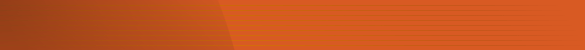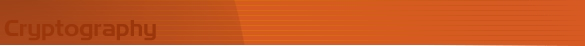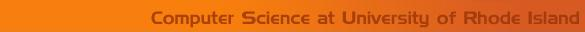Classical Cryptography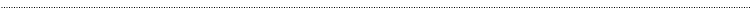Hill Cipher

Description

The Hill cipher is an example of a block cipher. A block cipher is a cipher in which groups of letters are enciphered together in equal length blocks. The Hill cipher was developed by Lester Hill and introduced in an article published in 1929.

Example

Encipher
In order to encrypt a message using the Hill cipher, the sender and receiver must first agree upon a key matrix A of size n x n. A must be invertible mod 26. The plaintext will then be enciphered in blocks of size n. In the following example A is a 2 x 2 matrix and the message will be enciphered in blocks of 2 characters.

Key Matrix: A =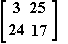Message: Mississippi

The first block MI corresponds to the matrix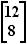. The sender will then calculate:

A=(mod 26)

The first two letters of the ciphertext correspond to 2,8 and are therefore CI. This step is repeated for the entire plaintext. If there are not enough letters to form blocks of 2, pad the message with some letter, say Z.

The message:

MI SS IS SI PP IK

will be enciphered as:

CI KK GE UW ER OY

Notice that the repeated digraphs IS, SS and repeated letters S and P in the plaintext are masked using the Hill cipher.

Decipher

To decipher a message, first calculate the inverse of the key A.(mod 26)

Then multiply the inverse of the key by each pair of ciphertext letters (mod 26) to recover the original text.

Key Matrix: A =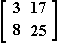Encrypted Message: CIKKGEUWEROY

A -1=(mod 26)